College Physics : Density, Specific Gravity, and Viscosity

Example Questions

Example Question #65 : College Physics

The reason why a coconut floats in water is because __________.

the density of the coconut is equal to the density of the water it displaces

the surface area of the coconut contacting the water is the same as the surface area of the water contacting the coconut

the gravitational force acting on the coconut is less than the buoyant force

the density of the coconut is less than the density of the water it displaces

the gravitational force acting on the coconut is less than the buoyant force

Explanation:

The equation for the buoyant force is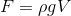. The gravitational force is constant. In order for the coconut to float, the buoyant force must be greater than the gravitational force. If the gravitational force is greater than the buoyant force, then the object will sink.

Example Question #71 : College Physics

A baseball has a mass of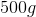, but it weighs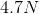when completely submerged in water. What is its volume assuming that the density of water is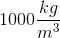?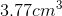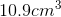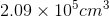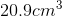Explanation:

We are given the mass of the baseball outside of the water. Using the weight equation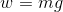with the gravitational constant being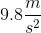and the mass being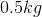, the weight of the baseball outside of the water is 4.905 N. (Be careful and convert the mass of the baseball from grams to kilograms since we are using SI units).

The buoyancy force is the difference of the weight of the baseball when it is in the air and when it is in the water. So subtract the two differences: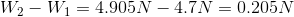Now we use the buoyancy equation: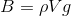whereis the buoyancy force,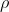is the density of water,is the volume of the baseball, and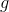is the gravitational constant. Plug in the known variables and solve for the volume.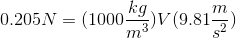and we get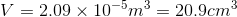All College Physics Resources